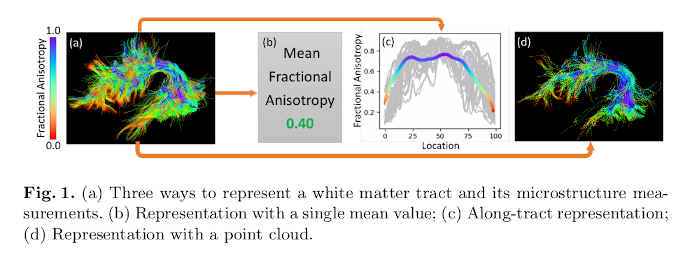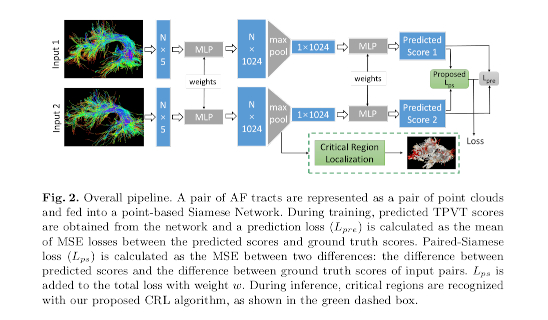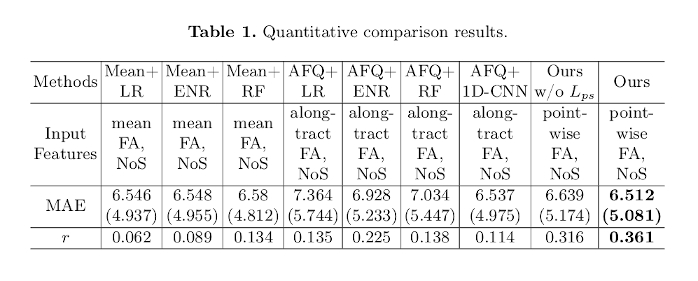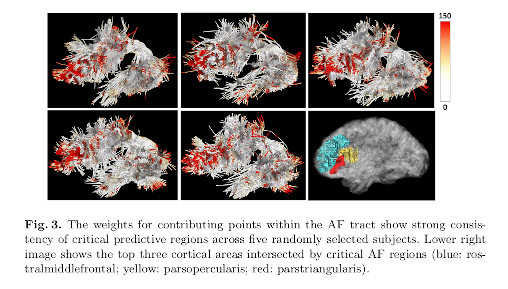# Introduction

Authors propose to use a point-based neural-network to predict the scores in a language comprehension and memory task using tractography data.# Methods

For each individual, the FA along the tracks and the number of streamlines are computed. The point spatial coordinates are augmented with these data.

Authors:

• Frame the problem as a regression task.
• Model streamlines as a point-cloud.
• Project diffusion-derived measures (FA) onto the streamline points.
• Use a point-based Siamese Network developed from PointNet.Use two loss terms:

• The $$L_{pre}$$: the MSE between the predicted score and the ground truth score. It is computed as the mean between the mentioned losses of the 2 inputs.
• The $$L_{ps}$$: Paired-Siamese Loss: the MSE between two differences: the difference between the two groun-truth scores, and the difference between the predicted scores.

The Critical Region Localization (CRL) method is also drawn from PointNet. The nuance is that they weight the contribution of the points by the number of max-pooled features in each considered segment.

## Data

806 HCP HYA dataset subjects; inlcuding their NIH Toolbox Picture Vocabulary Test (TPTV) scores.

Single shell UKF tractogramphy is used, and bundles are extracted with the WMA method. The left AF is selected predict the TPVT.

## Evaluation

• Mean Absolute Error (MAE)
• Pearson correlation coefficient between the predicted and ground truth scores

# Results# Conclusions

Authors:

• Propose a method to predict the performance on a language comprehension and memory task using dMRI tractography data.
• Propose a Critical Region Localization (CRL) method to localize informative anatomical regions along the track.
• Results are consistent across subjects, and localize regions that mostly contribute to the prediction.